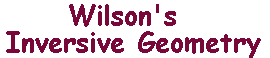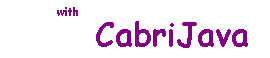Orthogonal Curves

 In angles between arcs, we looked at the signed angle between two arcs meeting at a point. Here, we will consider the magnitude of the angle(s) between two curves which meet at a point. As before, we define the angles in terms of the tangents at the point of intersection. Suppose that, in the sketch,